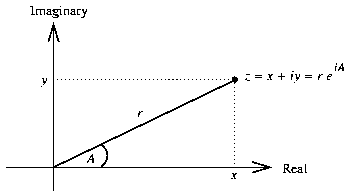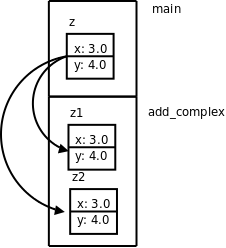1. 复合类型与结构体

1. 这门语言提供了哪些Primitive，比如基本类型，比如基本运算符、表达式和语句。

2. 这门语言提供了哪些组合规则，比如基本类型如何组成复合类型，比如简单的表达式和语句如何组成复杂的表达式和语句。

3. 这门语言提供了哪些抽象机制，包括数据抽象和过程抽象（Procedure Abstraction）struct complex_struct {
double x, y;
};

struct complex_struct {
double x, y;
} z1, z2;

struct complex_struct z3, z4;

struct {
double x, y;
} z1, z2;

#include <stdio.h>

int main(void)
{
struct complex_struct { double x, y; } z;
double x = 3.0;
z.x = x;
z.y = 4.0;
if (z.y < 0)
printf("z=%f%fi\n", z.x, z.y);
else
printf("z=%f+%fi\n", z.x, z.y);

return 0;
}

struct complex_struct { double x, y; };

int main(void)
{
struct complex_struct z;
...
}

struct complex_struct z = { 3.0, 4.0 };

Initializer中的数据依次赋给结构体的各成员。如果Initializer中的数据比结构体的成员多，编译器会报错，但如果只是末尾多个逗号则不算错。如果Initializer中的数据比结构体的成员少，未指定的成员将用0来初始化，就像未初始化的全局变量一样。例如以下几种形式的初始化都是合法的：

double x = 3.0;
struct complex_struct z1 = { x, 4.0, }; /* z1.x=3.0, z1.y=4.0 */
struct complex_struct z2 = { 3.0, }; /* z2.x=3.0, z2.y=0.0 */
struct complex_struct z3 = { 0 }; /* z3.x=0.0, z3.y=0.0 */

{}这种语法不能用于结构体的赋值，例如这样是错误的：

struct complex_struct z1;
z1 = { 3.0, 4.0 };

Initializer → 表达式
Initializer → { 初始化列表 }

（最后一个Designated-Initializer末尾可以有一个多余的,号）
Designated-Initializer → Initializer
Designated-Initializer → .标识符 = Initializer
Designated-Initializer → [常量表达式] = Initializer

Designated Initializer是C99引入的新特性，用于初始化稀疏（Sparse）结构体和稀疏数组很方便。有些时候结构体或数组中只有某一个或某几个成员需要初始化，其它成员都用0初始化即可，用Designated Initializer语法可以针对每个成员做初始化（Memberwise Initialization），很方便。例如：

struct complex_struct z1 = { .y = 4.0 }; /* z1.x=0.0, z1.y=4.0 */

struct complex_struct z1 = { 3.0, 4.0 };
struct complex_struct z2 = z1;
z1 = z2;

struct complex_struct add_complex(struct complex_struct z1, struct complex_struct z2)
{
z1.x = z1.x + z2.x;
z1.y = z1.y + z2.y;
return z1;
}

struct complex_struct z = { 3.0, 4.0 };1. z1初始化一个临时变量。

2. 函数返回并释放栈帧。

3. 把临时变量的值赋给变量z，释放临时变量。

 其实C99已经定义了复数类型_Complex。如果包含C标准库的头文件complex.h，也可以用complex做类型名。当然，只要不包含头文件complex.h就可以自己定义标识符complex，但为了尽量减少混淆，本章的示例代码都用complex_struct做标识符而不用complex

 我们在第 4 节 “结构体和联合体”会看到，结构体成员之间也可能有若干个填充字节。

 C99引入一种新的表达式语法Compound Literal可以用来赋值，例如z1 = (struct complex_struct){ 3.0, 4.0 };，本书不使用这种新语法。